# 手写 Java HashMap 核心源码

## HashMap 使用分析

``````//1 存
HashMap<String,String> map = new HashMap<>();
map.put("name","tom");

//2 取
System.out.println(map.get("name"));//输出 tom``````

## HashMap 原理分析

1 HashMap 是如何把 name,tom 存放的？ 下面我们用一张图来演示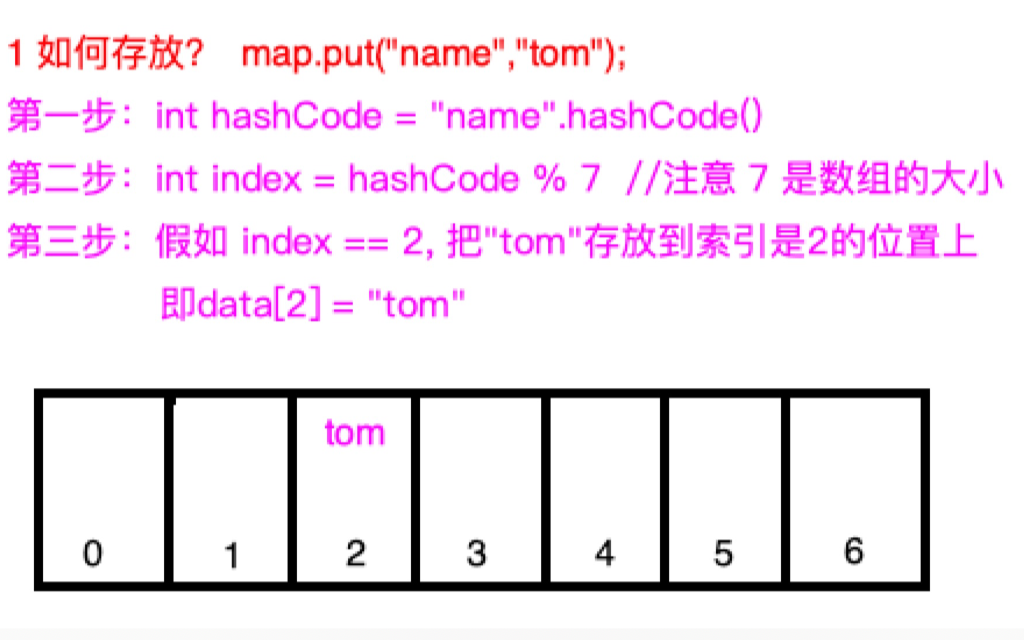1. 数组的大小是 7，那么数组的索引范围是 [0 , 6]
2. 取得 key 也就是 "name" 的 hashCode，这是一个数，不管这个数是多少，对 7 进行取余数，那么范围肯定是 [0 , 6]，正好和数组的索引是一样的。
3. "name".hashCode () % 7 的值假如为 2 ，那么 value 也就是 "tom" 应该存放的位置就是 2
4. data  = "tom" , 存到数组中。是不是很巧妙。

2 下面再来看看如何取？ 也用一张图来演示底层原理，如下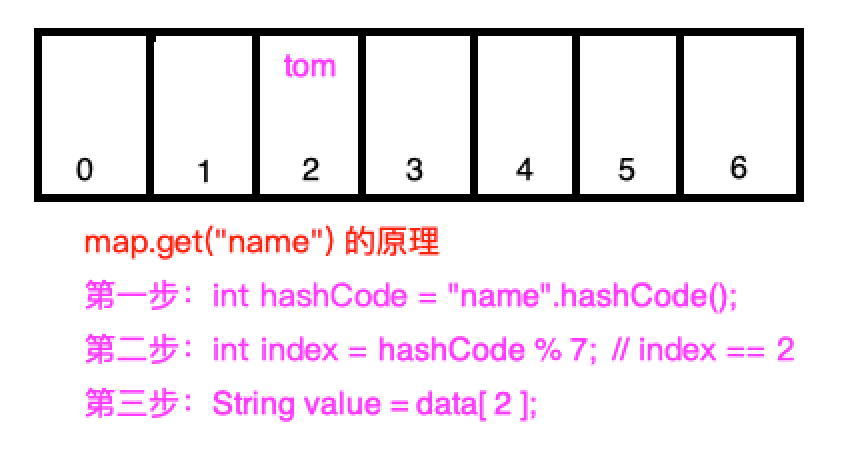1. 首先也是获取 key 也就是 "name" 的 hashCode 值
2. 用 hashCode 值对数组的大小 7 进行取余数，和存的时候运行一样，肯定也是 2
3. 从数组的第 2 个位置把 value 取出，即: String value = data 

1. 某个对象的 hashCode () 方法返回的值，在任何时候调用，返回的值都是一样的
2. 对一个数 n 取余数，范围是 [0, n - 1]

1. 存的时候，如果不同的 key 的 hashCode 对数组取余数，都正好相同了，也就是都映射在了数组的同一位置，怎么办？这就是 hash 冲突问题 比如 `9 % 7 == 2 ， 16 % 7 == 2` 都等于 2 答：数组中存放的是一个节点的数据结构，节点有 next 属性，如果 hash 冲突了，单链表进行存放，取的时候也是一样，遍历链表
2. 如果数组已经存满了怎么办？ 答：和 ArrayList 一样，进行扩容，重新映射
3. 直接使用 hashCode () 值进行映射，产生 hash 冲突的概论很大，怎么办？ 答：参考 JDK 中 HashMap 中的实现，有一个 hash () 函数，再对 hashCode () 的值进行运行一下，再进行映射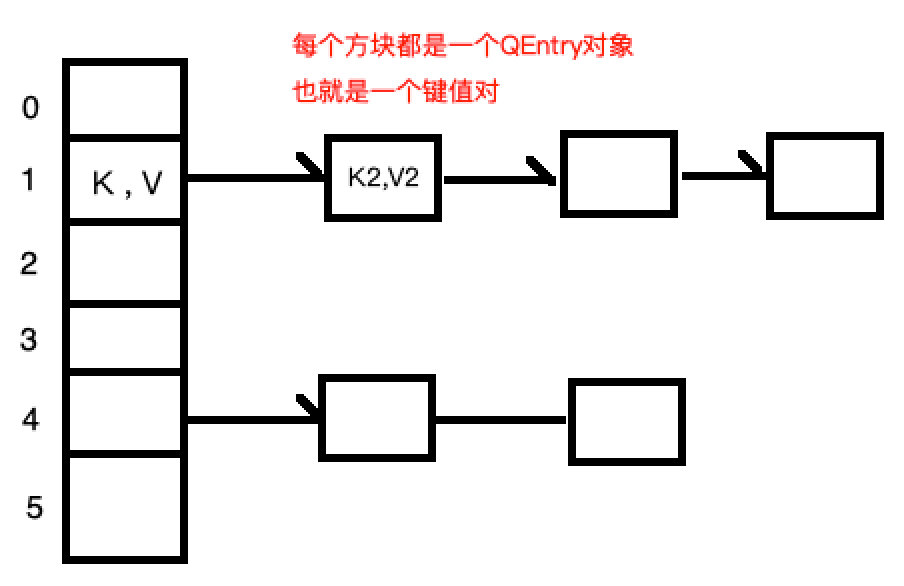## 手写 HashMap 核心源码

QEntry 的定义如下：

``````    //底层数组中存放的元素类
public static class QEntry<K, V> {
K key;      //存放key
V value;    //存放value
int hash;   //key对应的hash值

//hash冲突时，也就是映射的位置上已经有一个元素了
//那么新加的元素作为链表头，已经存放的放在后面
//即保存在next中，一句话：添加新元素时，添加在表头
QEntry<K, V> next;

public QEntry(K key, V value, int hash, QEntry<K, V> next) {
this.key = key;
this.value = value;
this.hash = hash;
this.next = next;
}
}``````

QEntry 类的定义有了，下面看下 QHashMap 类中需要哪些属性？ QHashMap 类的定义如下图：

``````public class QHashMap<K, V> {
//默认的数组的大小
private static final int DEFAULT_INITIAL_CAPACITY = 16;

//默认的扩容因子，当数据中元素的个数越多时，hash冲突也容易发生
//所以，需要在数组还没有用完的情况下就开始扩容
//这个 0.75 就是元素的个数达到了数组大小的75%的时候就开始扩容
//比如数组的大小是100，当里面的元素增加到75的时候，就开始扩容
private static final float DEFAULT_LOAD_FACTOR = 0.75f;

//存放元素的数组
private QEntry[] table;

//数组中元素的个数
private int size;

......
}    ``````

``````  public QHashMap() {
//创建一个数组，默认大小为16
table = new QEntry[DEFAULT_INITIAL_CAPACITY];

//此时元素个数是0
size = 0;
}``````

``````    /**
* 1 参数key,value很容易理解
* 2 返回V，我们知道，HashMap有一个特点，
* 如果调用了多次 map.put("name","tom"); map.put("name","lilei");
* 后面的值会把前面的覆盖，如果出现这种情况，返回旧值，在这里返回"tom"
*/
public V put(K key, V value) {
//1 为了简单，key不支持null
if (key == null) {
throw new RuntimeException("key is null");
}

//不直接用key.hashCode()，我们对key.hashCode()再作一次运算作为hash值
//这个hash()的方法我是直接从HashMap源码拷贝过来的。可以不用关心hash()算法本身
//只需要知道hash()输入一个数，返回一个数就行了。
int hash = hash(key.hashCode());

//用key的hash值和数组的大小，作一次映射，得到应该存放的位置
int index = indexFor(hash, table.length);

//看看数组中，有没有已存在的元素的key和参数中的key是相等的
//相等则把老的值替换成新的，然后返回旧值
QEntry<K, V> e = table[index];
while (e != null) {
//先比较hash是否相等，再比较对象是否相等，或者比较equals方法
//如果相等了，说明有一样的key,这时要更新旧值为新的value,同时返回旧的值
if (e.hash == hash && (key == e.key || key.equals(e.key))) {
V oldValue = e.value;
e.value = value;
return oldValue;
}
e = e.next;
}

//如果数组中没有元素的key与传的key相等的话
//把当前位置的元素保存下来
QEntry<K, V> next = table[index];

//next有可能为null，也有可能不为null，不管是否为null
//next都要作为新元素的下一个节点(next传给了QEntry的构造函数)
//然后新的元素保存在了index这个位置
table[index] = new QEntry<>(key, value, hash, next);

//如果需要扩容，元素的个数大于 table.length * 0.75 (别问为什么是0.75，经验)
if (size++ >= (table.length * DEFAULT_LOAD_FACTOR)) {
resize();
}

return null;
}``````

``````   //对hashCode进行运算，JDK中HashMap的实现，直接拷贝过来了
static int hash(int h) {
h ^= (h >>> 20) ^ (h >>> 12);
return h ^ (h >>> 7) ^ (h >>> 4);
}

//根据 h 求key落在数组的哪个位置
static int indexFor(int h, int length) {
//或者  return h & (length-1) 性能更好
//这里我们用最容易理解的方式，对length取余数，范围就是[0,length - 1]
//正好是table数组的所有的索引的范围

h = h > 0 ? h : -h; //防止负数

return h % length;
}``````

``````  //扩容，元素的个数大于 table.length * 0.75
//数组扩容到原来大小的2倍
private void resize() {
//新建一个数组，大小为原来数组大小的2倍
int newCapacity = table.length * 2;
QEntry[] newTable = new QEntry[newCapacity];

QEntry[] src = table;

//遍历旧数组，重新映射到新的数组中
for (int j = 0; j < src.length; j++) {
//获取旧数组元素
QEntry<K, V> e = src[j];

//释放旧数组
src[j] = null;

//因为e是一个链表，有可能有多个节点，循环遍历进行映射
while (e != null) {
//把e的下一个节点保存下来
QEntry<K, V> next = e.next;

//e这个当前节点进行在新的数组中映射
int i = indexFor(e.hash, newCapacity);

//newTable[i] 位置上有可能是null，也有可能不为null
//不管是否为null，都作为e这个节点的下一个节点
e.next = newTable[i];

//把e保存在新数组的 i 的位置
newTable[i] = e;

//继续e的下一个节点的同样的处理
e = next;
}
}

//所有的节点都映射到了新数组上，别忘了把新数组的赋值给table
table = newTable;
}``````

``````    //根据key获取value
public V get(K key) {

//同样为了简单，key不支持null
if (key == null) {
throw new RuntimeException("key is null");
}

//对key进行求hash值
int hash = hash(key.hashCode());

//用hash值进行映射，得到应该去数组的哪个位置上取数据
int index = indexFor(hash, table.length);

//把index位置的元素保存下来进行遍历
//因为e是一个链表，我们要对链表进行遍历
//找到和key相等的那个QEntry，并返回value
QEntry<K, V> e = table[index];
while (e != null) {

//比较 hash值是否相等
if (hash == e.hash && (key == e.key || key.equals(e.key))) {
return e.value;
}

//如果不相等，继续找下一个
e = e.next;
}

return null;
}``````

QHashMap 完整源码如下：

``````public class QHashMap<K, V> {
//默认的数组的大小
private static final int DEFAULT_INITIAL_CAPACITY = 16;

//默认的扩容因子，当数组的大小大于或者等于当前容量 * 0.75的时候，就开始扩容
private static final float DEFAULT_LOAD_FACTOR = 0.75f;

//底层用一个数组来存放数据
private QEntry[] table;

//数组大小
private int size;

//一个点节，数组中存放的单位
public static class QEntry<K, V> {
K key;
V value;
int hash;
QEntry<K, V> next;

public QEntry(K key, V value, int hash, QEntry<K, V> next) {
this.key = key;
this.value = value;
this.hash = hash;
this.next = next;
}
}

public QHashMap() {
table = new QEntry[DEFAULT_INITIAL_CAPACITY];
size = 0;
}

//根据key获取value
public V get(K key) {

//同样为了简单，key不支持null
if (key == null) {
throw new RuntimeException("key is null");
}

//对key进行求hash值
int hash = hash(key.hashCode());

//用hash值进行映射，得到应该去数组的哪个位置上取数据
int index = indexFor(hash, table.length);

//把index位置的元素保存下来进行遍历
//因为e是一个链表，我们要对链表进行遍历
//找到和key相等的那个QEntry，并返回value
QEntry<K, V> e = table[index];
while (e != null) {

//比较 hash值是否相等
if (hash == e.hash && (key == e.key || key.equals(e.key))) {
return e.value;
}

//如果不相等，继续找下一个
e = e.next;
}

return null;
}

/**
* 1 参数key,value很容易理解
* 2 返回V，我们知道，HashMap有一个特点，
* 如果调用了多次 map.put("name","tom"); map.put("name","lilei");
* 后面的值会把前面的覆盖，如果出现这种情况，返回旧值，在这里返回"tom"
*/
public V put(K key, V value) {
//1 为了简单，key不支持null
if (key == null) {
throw new RuntimeException("key is null");
}

//不直接用key.hashCode()，我们对key.hashCode()再作一次运算作为hash值
//这个hash()的方法我是直接从HashMap源码拷贝过来的。可以不用关心hash()算法本身
//只需要知道hash()输入一个数，返回一个数就行了。
int hash = hash(key.hashCode());

//用key的hash值和数组的大小，作一次映射，得到应该存放的位置
int index = indexFor(hash, table.length);

//看看数组中，有没有已存在的元素的key和参数中的key是相等的
//相等则把老的值替换成新的，然后返回旧值
QEntry<K, V> e = table[index];
while (e != null) {
//先比较hash是否相等，再比较对象是否相等，或者比较equals方法
//如果相等了，说明有一样的key,这时要更新旧值为新的value,同时返回旧的值
if (e.hash == hash && (key == e.key || key.equals(e.key))) {
V oldValue = e.value;
e.value = value;
return oldValue;
}
e = e.next;
}

//如果数组中没有元素的key与传的key相等的话
//把当前位置的元素保存下来
QEntry<K, V> next = table[index];

//next有可能为null，也有可能不为null，不管是否为null
//next都要作为新元素的下一个节点(next传给了QEntry的构造函数)
//然后新的元素保存在了index这个位置
table[index] = new QEntry<>(key, value, hash, next);

//如果需要扩容，元素的个数大于 table.length * 0.75 (别问为什么是0.75，经验)
if (size++ >= (table.length * DEFAULT_LOAD_FACTOR)) {
resize();
}

return null;
}

//扩容，元素的个数大于 table.length * 0.75
//数组扩容到原来大小的2倍
private void resize() {
//新建一个数组，大小为原来数组大小的2倍
int newCapacity = table.length * 2;
QEntry[] newTable = new QEntry[newCapacity];

QEntry[] src = table;

//遍历旧数组，重新映射到新的数组中
for (int j = 0; j < src.length; j++) {
//获取旧数组元素
QEntry<K, V> e = src[j];

//释放旧数组
src[j] = null;

//因为e是一个链表，有可能有多个节点，循环遍历进行映射
while (e != null) {
//把e的下一个节点保存下来
QEntry<K, V> next = e.next;

//e这个当前节点进行在新的数组中映射
int i = indexFor(e.hash, newCapacity);

//newTable[i] 位置上有可能是null，也有可能不为null
//不管是否为null，都作为e这个节点的下一个节点
e.next = newTable[i];

//把e保存在新数组的 i 的位置
newTable[i] = e;

//继续e的下一个节点的同样的处理
e = next;
}
}

//所有的节点都映射到了新数组上，别忘了把新数组的赋值给table
table = newTable;
}

//对hashCode进行运算，JDK中HashMap的实现，直接拷贝过来了
static int hash(int h) {
h ^= (h >>> 20) ^ (h >>> 12);
return h ^ (h >>> 7) ^ (h >>> 4);
}

//根据 h 求key落在数组的哪个位置
static int indexFor(int h, int length) {
//或者  return h & (length-1) 性能更好
//这里我们用最容易理解的方式，对length取余数，范围就是[0,length - 1]
//正好是table数组的所有的索引的范围

h = h > 0 ? h : -h; //防止负数

return h % length;
}

}``````

`````` public static void main(String[] args) {
QHashMap<String, String> map = new QHashMap<>();
map.put("name", "tom");
map.put("age", "23");
String oldValue = map.put("address", "shanghai"); //key一样，返回旧值，保存新值

System.out.println(map.get("name"));
System.out.println(map.get("age"));

System.out.println("旧值=" + oldValue);
}``````

``````tom
23Souleigh ✨
2年前九路
2年前
1 手写ArrayList核心源码九路
2年前
3 手写Java HashMap核心源码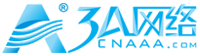3A网络
11个月前九路
2年前
4.1 手写Java PriorityQueue 核心源码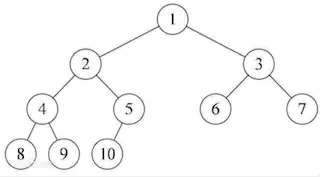九路
2年前
4.2 手写Java PriorityQueue 核心源码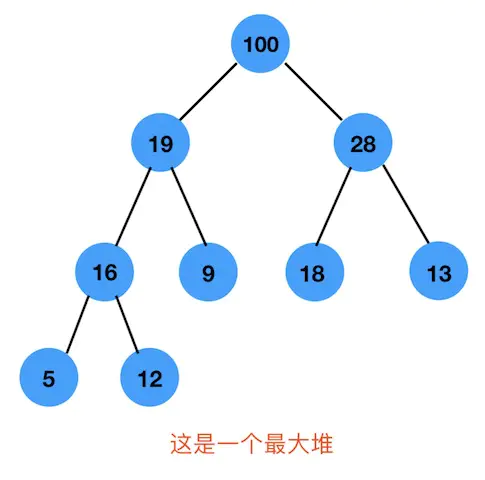九路
2年前
5 手写Java Stack 核心源码
Stack是Java中常用的数据结构之一，Stack具有"后进先出(LIFO)"的性质。只能在一端进行插入或者删除，即压栈与出栈栈的实现比较简单，性质也简单。可以用一个数组来实现栈结构。1.入栈的时候，只在数组尾部插入2.出栈的时候，只在数组尾部删除我们来看一下Stack的用法：如下publicstaticvoidmai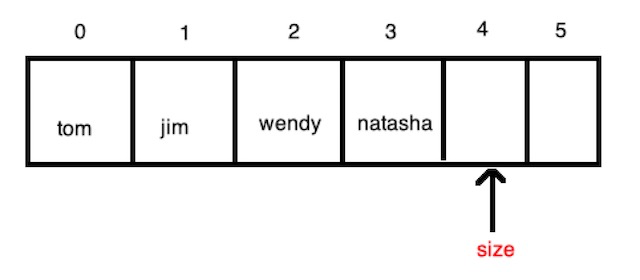九路
2年前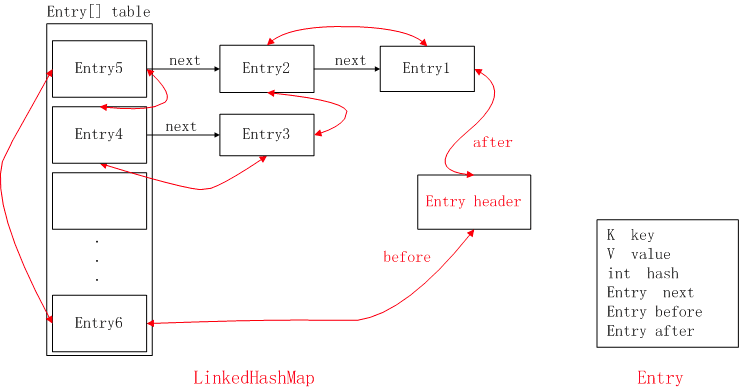九路
2年前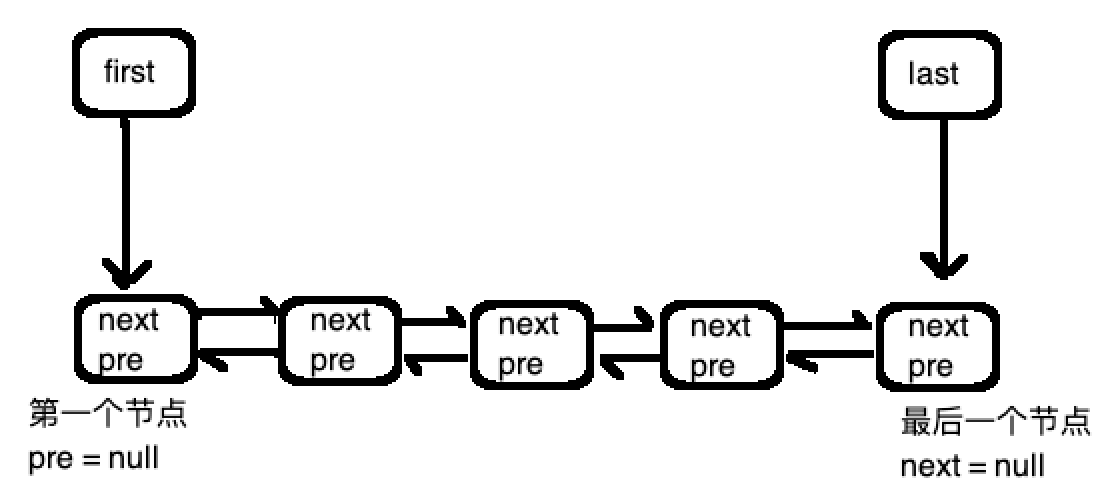Easter79
1年前
Spring核心源码3A网络
Lv1

58

5

36